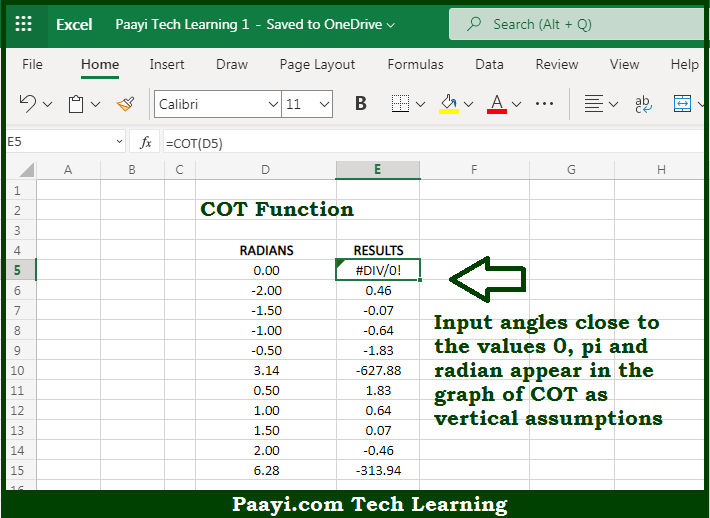# Learn How to Use Microsoft Excel COT Function

Written by | 0 Comments | 574 Views

In this article, you will learn how to use the Microsoft Excel COT function and its prime function in Microsoft Excel. You will also get to know the Microsoft Excel COT function return value and syntax with the help of some examples.

Microsoft Excel COT Function

The main purpose of the Microsoft Excel COT function is to get the cotangent of the angle. That implies, with the help of the COT function you can able to return the cotangent of the angle provided in radians. In geometry, the cotangent of an angle returns the ratio of the length of the adjacent side over the length of the opposite side of the corresponding right triangle. So, with the help of the COT function, you can able to get the cotangent of the provided angle.

Return Value of COT Function

The return value will be the cotangent value.

Syntax of COT Function

=COT(number)

Where the arguments:

• number: This is the angle provided in radians.

## How to Use Microsoft Excel COT Function?So we know that Microsoft Excel COT function you can able to get the cotangent of the angle. That implies, with the help of the COT function you can able to return the cotangent of the angle provided in radians. In geometry, the cotangent of an angle returns the ratio of the length of the adjacent side over the length of the opposite side of the corresponding right triangle. So, with the help of the COT function, you can able to get the cotangent of the provided angle.